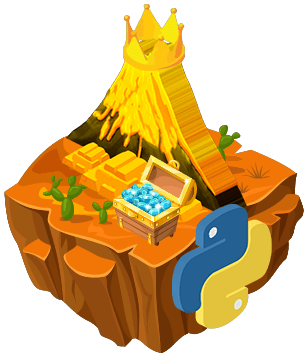Master Python

Be familiar with Python arrays and functions.

## 描述

We make a great team! I am having a blast here! How about we collect some more gifts?  Just remember, we need to use the fewest blocks we can and we need to stay the course - no wondering around!

## 任務

Add each number on the red cell to the corresponding number on the green cell (number in the same row). Print the results onto the yellow cell of the same row.

Hint: The numbers will change after each run. You should read  from cells.

## A lot of multiplications!

Multiply each number on the red cells to the corresponding number on the green cells (number in the same row). Print the results onto the yellow cells of the same row.

Hint: The numbers will change after each run. You should read  from cells.

## Result of addition is known!

This is different. We have the results but we are missing the second set of numbers. Can you print the missing numbers onto the yellow cells to complete the equation?

Hint: The numbers will change after each run. You should read  from cells.

## Reverse multiplication

We have the results but we are missing the second set of numbers. Can you print the missing numbers onto the yellow cells to complete this multiplication problem?

Hint: The numbers will change after each run. You should read  from cells.

## Subtraction list!

The first set of numbers for this subtraction equation is missing. Can you calculate it?

Hint: The numbers will change after each run. You should read  from cells.Master Python

Be familiar with Python arrays and functions.隱私政策使 使用條款